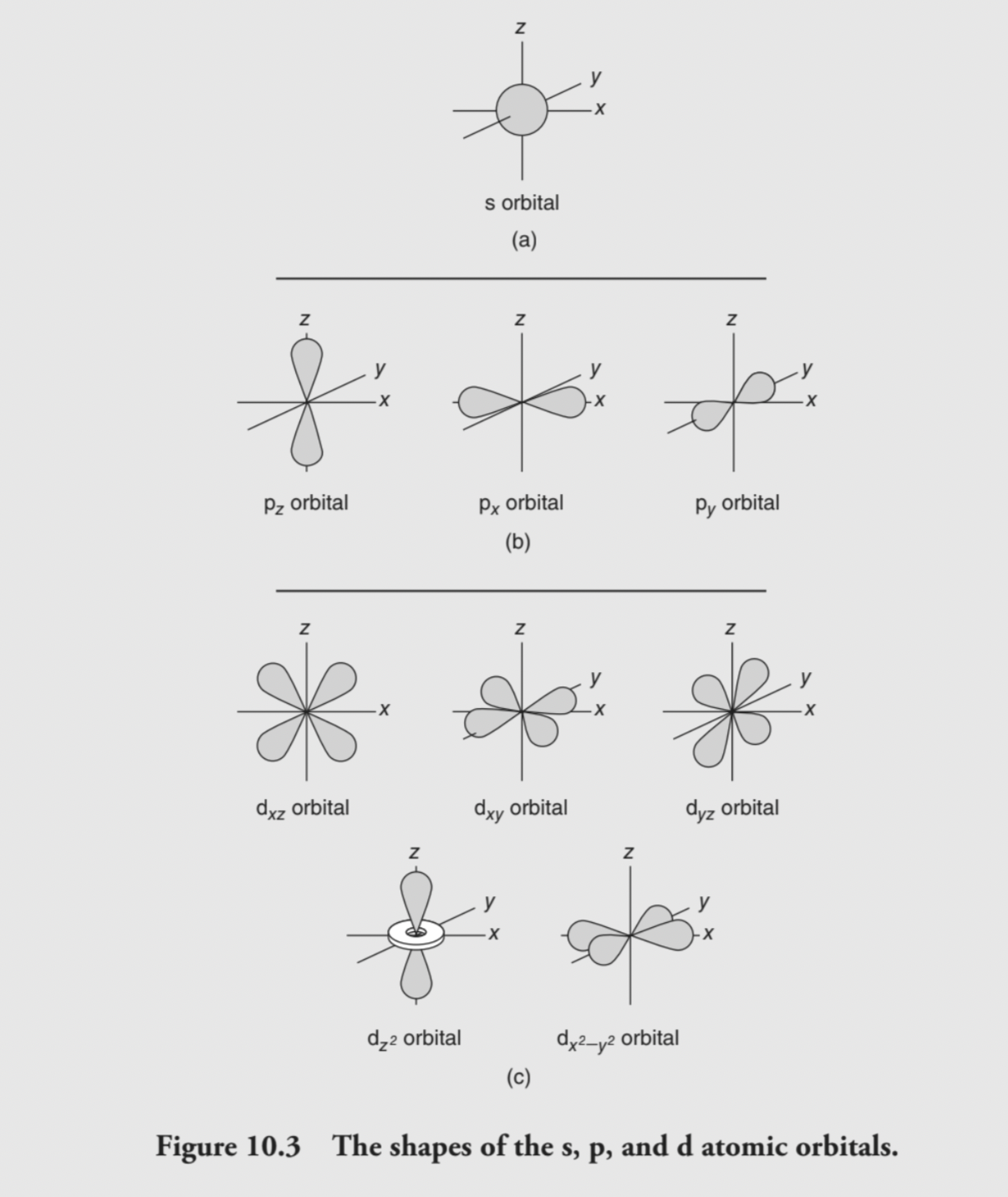# THE NATURE OF LIGHT

• Electromagnetic spectrum- radiant energy composed of gamma rays, x-rays, ultraviolet light, visible light,etc

• Frequency-- the number of waves that pass a point per second

• Wavelength is the distance between two identical points on a wave

• Amplitude is the height if the wave and is related to the intensity amd amplitude of a wave

• Speed light all electromagnetic radiation travels about the same speed in a vacuum 3.0 x 10^8

# WAVE PROPERTIES OF MATTER

• equation l = h/mv, which indicates a mass (m) moving with a certain velocity (v) would have a specific wavelength (l) associated with it.

# ATOMIC SPECTRA

• line spectrum, a series of fine lines of colors, instead of a continuous spectrum like a rainbow.

• The Bohr model postulated a ground state for the electrons in the atom, an energy state of lowest energy, and an excited state, an energy state of higher energy.

# ATOMIC ORBITALS

• Schrödinger developed a mathematical description of the electron’s motion called a wave function or atomic orbital.

• Squaring the wave function (orbital) gives the volume of space in which the probability of finding the electron is high. This is commonly referred to as the electron cloud.

• Schrödinger’s equation required the use of three quantum numbers to describe each electron within an atom, corresponding to the orbital size, shape, and orientation in space.

• The first quantum number is the principal quantum number (n).

• The smaller the value of n, the closer the orbital is to the nucleus. The number n is sometimes called the atom’s shell.

• The second quantum number is the angular momentum quantum number (l). Its value is related to the principal quantum number and has allowed values of 0 up to (n - 1).

• These are• The third quantum number is the magnetic quantum number (ml). It describes the orientation of the orbital around the nucleus.

• The fourth quantum number, the spin quantum number (ms), indicates the direction the electron is spinning.

# Photoelectron (Photoemission) Spectroscopy (PES)

• Photoelectron spectroscopy is one of a group of related techniques where high-energy photons remove an electron from an atom in a photoelectric effect process.

• The kinetic energy is equal to the energy of the photon minus the binding energy of the electron. The binding energy is the energy holding the electron in the atom and can be rather difficult to measure.

• X-ray photons can excite core electrons.

In compounds, other atoms bonded to the atom of interest can influence the effective nuclear charge**.**